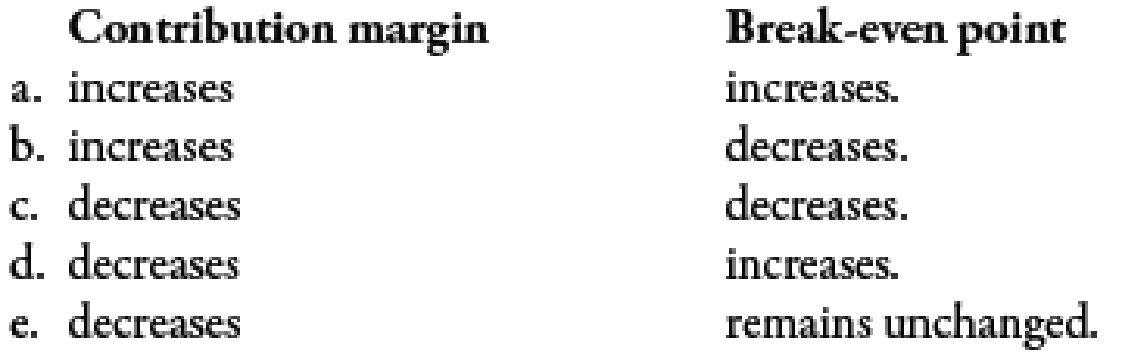Chapter 7, Problem 1MCQ### Managerial Accounting: The Corners...

7th Edition
Maryanne M. Mowen + 2 others
ISBN: 9781337115773

#### Solutions

Chapter
Section### Managerial Accounting: The Corners...

7th Edition
Maryanne M. Mowen + 2 others
ISBN: 9781337115773
Textbook Problem
5 views

# If the variable cost per unit goes down,To determine

Identify the effect of a decrease in variable cost per unit on contribution margin and break-even analysis.

Explanation

Variable Cost Per Unit:

Variable cost per unit can be computed by dividing the total variable cost by the number of units or by adding all the variable costs per unit. Variable costs can be defined as the costs which increase with the increase in output and decrease with the decrease in output.

b.

Contribution margin increases due to decrease in variable cost which in turn decreases the break-even point. Therefore, option b is the correct answer.

a.

Contribution margin and break-even point are inversely related to each other. If one increases, then the other will decrease...

### Still sussing out bartleby?

Check out a sample textbook solution.

See a sample solution

#### The Solution to Your Study Problems

Bartleby provides explanations to thousands of textbook problems written by our experts, many with advanced degrees!

Get Started

#### Find more solutions based on key concepts